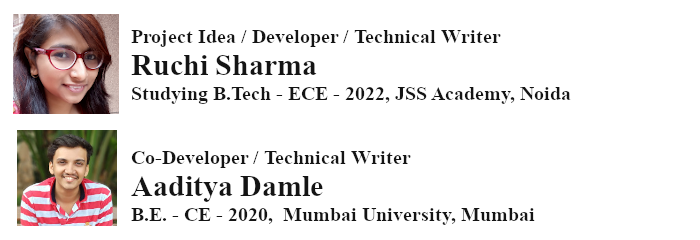| Technical Review: ABCOM Team | Level: Intermediate | Banner Image Source : Internet |

# Overview

We all are going through a pandemic and because of the spread of this coronavirus, more than a million people have already lost their lives across the world. Economy is affected because of lockdowns. With Unlock now in effect, it is our responsibility to take precautions so that another cycle does not start. There is a famous saying “Prevention is better than cure” and a famous quote:

“An ounce of prevention is worth a pound of cure”. - Benjamin Franklin

Everyone is accepting new habits like wearing a mask, using sanitizer etc. as a new normal. In this huge society, you do find exceptions - people not wearing masks may come close enough to you. In this tutorial, you will learn how to develop a machine learning model that monitors people approaching you and warns you instantly when persons not wearing masks come in proximity.

This demo video will give you a brief idea about the working of this project.

In the video, you can see that the program first detects my face and marks it with a rectangle in green color. Wearing a mask, when I approach the camera, it generates no warning. However, approaching the camera without a mask generates an alert in red color when I am close enough to the camera. You will be able to control the closeness in your code.

# Trying it on Your Machine

Before I explain the program working and the entire code, it will be worthwhile to try the above demo on your machine. You will need to install a few packages to run the demo. Install the following packages from the links provided (I assume that you already have Python installed on your machine):

``````python warn.py
``````

This opens a webcam view on your screen. You can now try the application by moving towards the webcam from a distance, with and without a mask. To adjust the range for warning, you will need to set a variable in warn.py that I will show you when I discuss the project code.

Now that you have successfully installed the application and understood its working, I will explain to you how it works. Later on, I will also explain the code for creating and training the machine learning model used in warn.py.

# Project Description

To detect a mask on a face, you first need to detect face. For face detection, we will use OpenCV. For detecting a mask on the face, we will use a dataset provided in the Kaggle competition. We will create and train a neural network model for mask detection and as said earlier, we will save this into a .h5 file. We use this .h5 file in warn.py for real-time mask detection. To develop a neural network model, we will use the technique of transfer learning where we use a pre-trained image classifier and add a layer on top of it for mask detection. Let me now explain the warn.py file. After I do this, I will explain to you the model training code.

The warn.py file contains the code for mask detection and warning generation. We import the following libraries:

``````from tensorflow.keras.applications.mobilenet_v2 import preprocess_input
from tensorflow.keras.preprocessing.image import img_to_array
import numpy as np
import cv2
``````

The pre-trained image classifier is available in the TensorFlow library. They call it mobilenet_v2, which we have imported in the above code. The mobilenet_v2 is a convolution neural network (CNN) and is 53 layers deep. This pre-trained model can classify 1000 types of objects. The webcam camera captured images need some preprocessing to convert them into a format required by this trained model. We use the `preprocess_input` function provided in the library for this purpose. For converting the image to a tensor we use `img_to_array`. For loading a trained model, we use the `load_model` of TensorFlow library. We add a few layers on top of the pre-trained model and train it further for detecting faces with and without masks. The model training code is provided in Mask_Detector.ipynb project, which is discussed later. We use cv2 for real-time image capture.

After completing the required imports, we develop a function for detecting masks.

We declare the function as follows:

``````def mask_detection(real_T_vid, Net, MODEL):
``````

The function takes three parameters:

• real_T_vid - we use this for passing the real time video stream to the function
• Net - it will read the data of face detection models. This face detection model will help us detect faces in real time video streams.
• MODEL - our pre-trained model is passed through this parameter.

In the function body, first we extract the window height and width of the video stream.

``````    (h,w)=real_T_vid.shape[:2]
``````

Next, we extract blobs from the video stream for further image processing.

## Creating, Processing Blobs

We use `blobFromImage` function provided by opencv for extracting a blob.

``````blob=cv2.dnn.blobFromImage(real_T_vid, 1.0, (224, 224),(104.0, 177.0, 123.0))
``````

The function performs mean subtraction of RGB values and the image scaling. The first parameter to the function is the video stream. The second parameter determines the scaling. This is set to 1.0 in our case, so the original image is retained as is - not scaled. The parameter (224, 224) indicates the size to which we resize the image - the mobilenet network was trained on images having size 224x224 pixels. The last parameter (104.0, 177.0, 123.0) is a tuple of mean subtraction values (R,G,B). We subtract these mean values from image data for normalization. You can get further details on this method in the link provided in the references section.

We use the `setInput` function of OpenCV to pass the blob as an input to our network of detecting faces. You can study the other attributes of this function in `setInput` documentation.

``````Net.setInput(blob)
``````

After setting the input to `Net` we call its forward function to detect the faces in the block and get their locations in the image.

``````detections= Net.forward()
``````

This reference (Net class reference) provides further information on this function.

Next, we declare three arrays for our further use:

• Faces - used for storing detected faces
• Location - the location of the box that surrounds a face. This is the bounding rectangle that you observed in the demo video.
• Prediction - stores the model’s prediction, whether or not the face is masked.
``````faces=[]
location=[]
prediction=[]
``````

## Processing Detections

We set up a loop for processing the detected faces in the blob:

``````for i in range(0, detections.shape):
``````

For each face, we extract the confidence level of our model’s face detection.

``````for i in range(0, detections.shape):
confidence= detections[0,0,i,2]
``````

If confidence is greater than 0.5 we draw a box around the face.
Observe a green box around my face in the image below.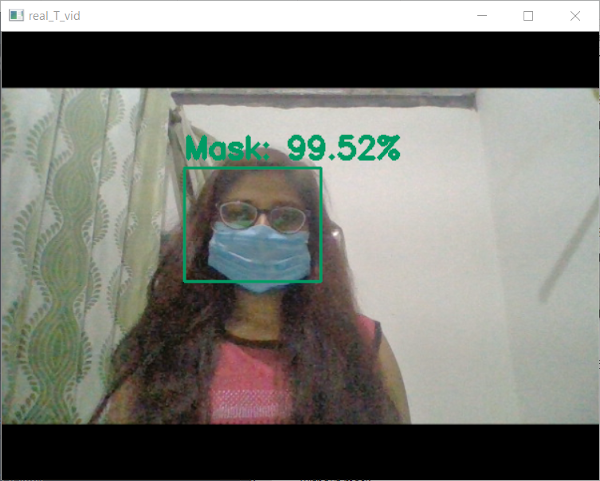``````if confidence>0.5:
box= detections[0,0,i,3:7]*np.array([w,h,w,h])
(X1,Y1,X2,Y2)=box.astype("int")
(X1, Y1) = (max(0, X1), max(0, Y1))
(X2, Y2) = (min(w-1, X2), min(h-1, Y2))
``````

The `X1`,`Y1`,`X2`,`Y2` are the corners of this box.

We create a `face` variable using these coordinates to mark out a detected face in the video.

``````face = real_T_vid[Y1:Y2, X1:X2]
``````

## Preprocessing Image

The image captured by `cv2` is in BGR format. We need to convert it to RGB before we preprocess it.

``````face = cv2.cvtColor(face, cv2.COLOR_BGR2RGB)
``````

Resize the image:

``````face = cv2.resize(face, (224, 224))
``````

Convert all the image data into an array and preprocess it using the built-in library.

``````face = img_to_array(face)
face = preprocess_input(face)
``````

## Store Face and its Location

Add the detected face and the location into our previously declared arrays.

``````faces.append(face)
location.append((X1,Y1,X2,Y2))
``````

If the faces array contains any elements, convert the image data into a numeric array and pass it to our model to detect if the face has a mask or not. We use the model's `predict` method to do the prediction. We return the face location and the prediction to the caller.

``````    if len(faces)>0:
faces = np.array(faces, dtype="float32")
prediction = MODEL.predict(faces, batch_size=32)
return (location, prediction)
``````

After developing the function for mask detection as above, we will now proceed to start our webcam for some real time mask detections. First, we will load the two models - for face and mask detections.

``````proto_path = r"deploy.prototxt"
weight_path = r"res10_300x300_ssd_iter_140000.caffemodel"
``````

The mask detection model is developed by us. The model development code is discussed later. We load our trained model as follows:

``````MODEL = load_model("mask_detector.model")
``````

## Capturing Video

We start the real time video capture using the built-in webcam by calling the `VideoCapture` method of OpenCV.

``````video = cv2.VideoCapture(0)
``````

We define an infinite loop for video capture which will be terminated when the user presses ‘`q`’ on his keypad.

``````while True:
``````

The `real_T_vid` is the window name in which the video is displayed. The video is captured by calling the `read` method:

``````    _, real_T_vid = video.read()
``````

We resize the video frame to a width of 600 pixels maintaining the aspect ratio.

``````    ht,wd = real_T_vid.shape[:2]
real_T_vid = cv2.resize(real_T_vid, dsize=(600,ht))
``````

We call our earlier defined `mask_detection` function to capture the location of a face and the model’s prediction on it.

``````    (location, prediction) = mask_detection(real_T_vid, Net, MODEL)
``````

To mark the faces along with the bounding rectangles and the mask/no-mask prediction, we iterate through our collection:

``````    for (box, pred) in zip(location, prediction):
(X1, Y1, X2, Y2) = box
``````

We create a colored label depending on whether the mask prediction is greater than 75%.

``````        label = "Mask" if mask > .75 else "No Mask"
color = (100, 155, 0) if label == "Mask" else (0, 100, 155)
``````

The two images below show the bounding box and the predictions.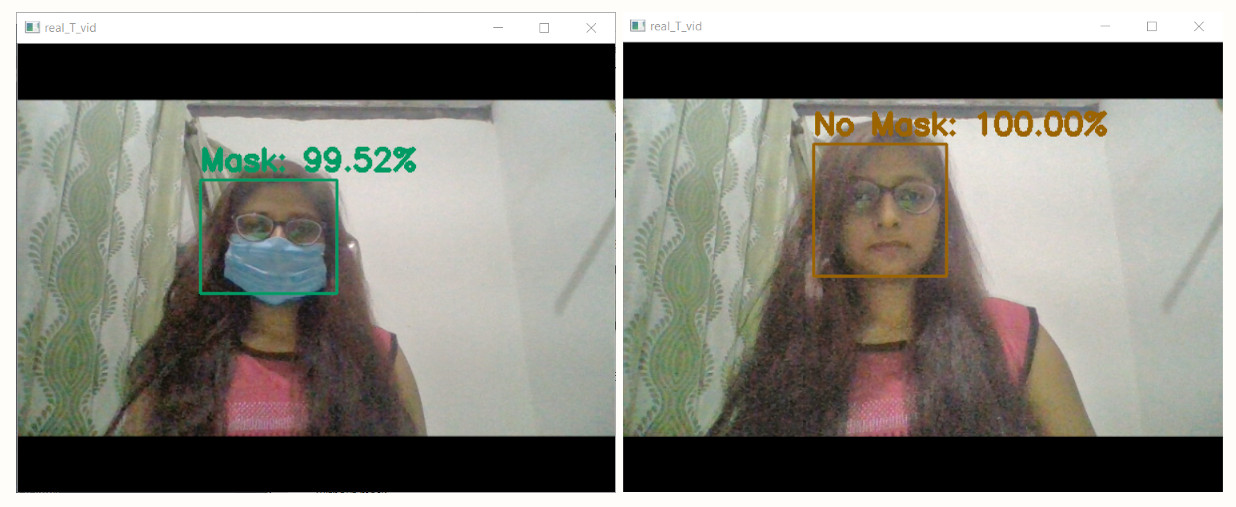## Condition to Maintain Social Distancing

We display an alert if the approaching person is not wearing a mask and is too close to the webcam. We check the width of the detected face. If this is greater than 60, we create a closeness warning error. Note that the width of our video frame was set earlier to 600. It means, if the detected face is 60/600, i.e. 1/10th the image size, the object is close to the camera. Change this constant to match your distance requirement for a warning.

``````       # To define the distance from camera, change the constant
# value 60 in the following if condition
if X2-X1>(60):
# Check if prediction for without mask is 75% and above
cv2.putText(real_T_vid,'Maintain Distance',(X2, Y2-5),cv2.FONT_HERSHEY_SIMPLEX,1,(0,0,255),2)
# print("Warning")
cv2.putText(real_T_vid, label, (X1, Y1 - 10),cv2.FONT_HERSHEY_SIMPLEX, 1, color, 3)
cv2.rectangle(real_T_vid, (X1, Y1), (X2, Y2), color, 2)
``````

The following screenshot shows the condition when an alert is generated.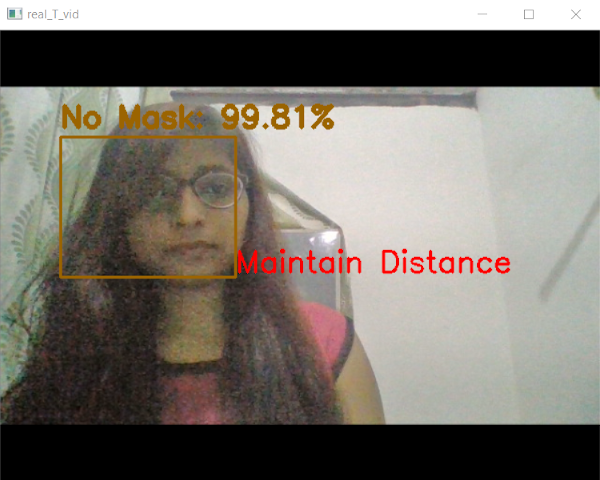We now display the video capture window to the user.

``````    cv2.imshow("real_T_vid", real_T_vid)
``````

The loop terminates when the user clicks ‘q’.

``````    key = cv2.waitKey(1) & 0xFF
# if the `q` key was pressed, break from the loop
if key == ord("q"):
break
``````

We now close all the open windows of our application and stop the video.

``````cv2.destroyAllWindows()
video.stop()
``````

Now, I will discuss the code used for training a neural network model for mask detection.

The full source for creating and training the model for detecting face masks is available in a Colab project (Mask_Detector.ipynb) in your downloaded source. We will use TensorFlow 2 for model development. I used Colab for the model development to take advantage of the GPU provided by Google for training. If you are new to Colab, then check out this short video tutorial on Google Colab.

Import the following packages:

``````import tensorflow
from tensorflow import keras
from sklearn.preprocessing import LabelBinarizer
from sklearn.model_selection import train_test_split
import numpy as np
import os
``````

``````!wget https://github.com/abcom-mltutorials/mask_detector/archive/master.zip
``````

The file downloaded from this link is in compressed format. Use the unzip command to extract the dataset as shown below.

``````! unzip master.zip
``````

## Organizing Data

``````path_dir = "/content/mask_detector-master/data"
``````

We have two categories one is with a mask and another one is without a mask.

``````categories = ["with_mask", "without_mask"]
``````

Create two arrays for storing a dataset of images along with its label with mask and without mask.

``````dataset = []
label = []
``````

Then concatenate the path directory for each category in categories we have defined above using the os.path.join method.

``````for category in categories:
path = os.path.join(path_dir, category)
``````

Then create a list of all the images that are stored in the mentioned directory of our dataset using `os.listdir` method. Concatenate the path directory of each image of our dataset using `os.path.join` method.

``````for img in os.listdir(path):
img_path = os.path.join(path, img)
``````

Now we have a list of all the images that are concatenated with their respective path. Load the image using `load_img` method and resize them to the size required by the pre-trained model.

``````Img = keras.preprocessing.image.load_img(img_path, target_size=(224, 224))
``````

Convert list of images to an array using `img_to_array` method. This converts the image to a numpy array, and this method returns a 3D array.

``````Img = keras.preprocessing.image.img_to_array(Img)
``````

We preprocess these images using the following command:

``````Img = keras.applications.mobilenet_v2.preprocess_input(Img)
``````

We will store the complete array of images in our already declared array named `dataset.` And we will append the array of labels with all the labels of our dataset as for both categories, mask and without_mask.

``````dataset.append(Img)
label.append(category)
``````

## Converting Categories into Binary

Now convert both categories into binary format like 0,1 using label binarizer.

``````label_binarizer = LabelBinarizer()
``````

Transform our declared array of labels from multi-class labels to binary labels using the `fit_transform` method.

``````label = label_binarizer.fit_transform(label)
``````

Then convert a class vector (integers) to binary class matrix using `to_categorical`.

``````label = keras.utils.to_categorical(label)
``````

## Create Arrays of Dataset and Labels

Convert the dataset array to a matrix of float type and convert array of labels into matrix of labels.

``````dataset = np.array(dataset, dtype="float32")
label = np.array(label)
``````

## Split Dataset into Training and Testing

Divide the dataset along with labels for training and testing. I am using here a test size of 20% and a train size of 80% which means 20% data will be allocated to the test set. `Random_state` is for shuffling the data before splitting it. Set X and Y for two categories.

``````(trainX, testX, trainY, testY) = train_test_split(dataset, label,
test_size=0.20, stratify=label, random_state=42)
``````

## Apply Augmentation on Images

We are using `ImageDataGenerator` to apply augmentation and increase variation in the dataset by various operations like shift, rotate, zoom, shear, horizontal flip etc. Because of these operations some space is generated between the border of image and actual size of image. Nearest is default `fill_mode` in which it fills border pixels in this empty space.

You can read about its other arguments (image data generator) from the reference section.

``````data_augmentation = keras.preprocessing.image.ImageDataGenerator(
rotation_range=20,
zoom_range=0.07,
width_shift_range=0.2,
height_shift_range=0.2,
shear_range=0.10,
horizontal_flip=True,
fill_mode="nearest")
``````

# Model creation

When the size of the dataset is less, we cannot use simple CNN despite getting good accuracy because the model can fail if the testing set is incongruous with the training set. Pre-trained models withstand such scenarios and thus they are used when the size of the dataset is small and you need good accuracy in your model. When you use a pre-trained model into your model definition, you are taking advantage of somebody else’s training and we call this transfer learning.

Use the following code to define the `pre_trained_model`.We have used `MobileNetV2` from keras. We specify weights from pre-trained model to imagenet. The `include_top` parameter is set to false for fully connected layers and we set the input shape i.e. the desired image shape in the format (height, width, channel (3 for RGB)).

``````pre_trained_model = keras.applications.MobileNetV2(weights="imagenet", include_top=False,
input_tensor=keras.layers.Input(shape=(224, 224, 3)))

for layer in pre_trained_model.layers:
layer.trainable = False
``````

## Sequential Model

We use a sequential model from Keras to join the pre-trained model defined above. The Sequential groups a linear stack of layers into a model. The sequential API allows you to create models layer-by-layer for most problems.

``````model = tensorflow.keras.Sequential(
[keras.Input(shape=(224, 224, 3)),
pre_trained_model,
keras.layers.MaxPooling2D(pool_size=(2,2)),
keras.layers.Dense(720, activation="relu"),
keras.layers.Dense(32, activation="relu"),
keras.layers.Dropout(0.3),
keras.layers.Flatten(),
keras.layers.Dense(units=2,activation="softmax")
]
)
``````

The first layer specifies shape followed by the `pre_trained_model`. Then we use a pooling layer. After this we will use two dense layers. We use dense for fully connected layers. In this layer, hidden layers are completely connected so they can combine features of previous layers very well. The first and second layers contain 720 and 32 units in the hidden layer respectively with ReLU activation. Then, I added a dropout layer of 30%. We use dropout to avoid overfitting. Then we add a Flatten layer to convert data into 1-D array format. Finally, we define the output dense layer and we have selected 2 units as we have 2 categories.

Build and print the model summary using following code:

``````model.build([None, 224, 224, 3])
model.summary()
``````

The output is shown below: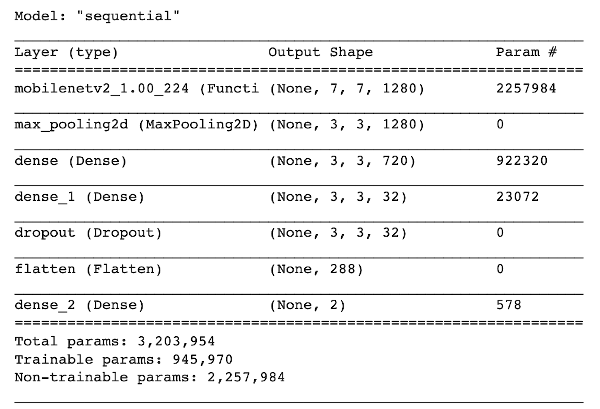## Compiling Model

Compile the model using following command:

``````    model.compile(loss="binary_crossentropy",
metrics=["accuracy"])
``````

## Model Training

After compiling the model, we will now train the model by calling its fit method. As in any other fit method, first we include augmentation, steps per epoch which defines the length of each epoch, the validation data which enables the `val_loss` and `val_acc` during each epoch and the validation steps which are defined during initialization. Also initialize the epochs and batch size before calling fit method.

``````EPOCHS =10
batchSize = 40
FIT = model.fit(
data_augmentation.flow(trainX, trainY, batch_size=batchSize),
steps_per_epoch=len(trainX) // batchSize,
validation_data=(testX, testY),
validation_steps=len(testX) // batchSize,
epochs= EPOCHS)
``````

The figure below shows the last 5 epochs of the model training. As you can observe that the model has a validation accuracy greater than 99%. This is a good sign to confirm that our model has trained properly.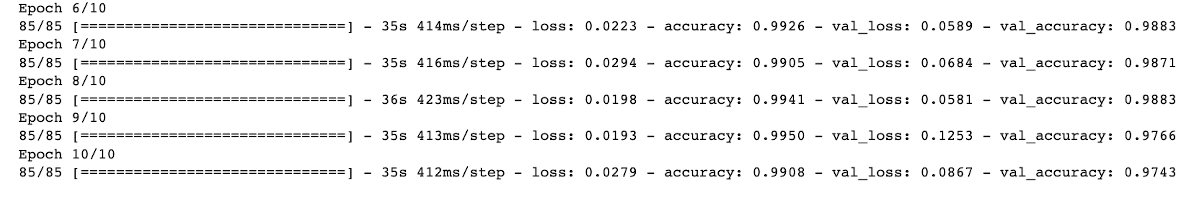## Inference

We make predictions on the test set by calling its predict method.

``````PREDICT = model.predict(testX, batch_size=batchSize)
``````

Make two lists to contain max values for predicted and actual test values. We will use the argmax method to return indices of maximum value.

``````pred_class = []
ac_class = []
for i in range(len(PREDICT)):
pr = PREDICT[i].argmax()
pred_class.append(pr)
ac = testY[i].argmax()
ac_class.append(ac)
``````

Then make 2 lists which will contain the predicted and actual output results gained from comparing max values in the above code with allotted strings.

``````output = {0:"with_mask", 1:"wihout_mask"}
pred_op = []
ac_op = []
for i in range(len(pred_class)):
pred_op.append(output[pred_class[i]])
ac_op.append(output[ac_class[i]])
``````

Finally, create and increment the counter if the actual and predicted values match. Then calculate accuracy of the first batch in percentage.

``````correct_pred = 0
for i in range(batchSize):
if pred_op[i] == ac_op[i]:
correct_pred += 1
``````

Output is as follows;

``````Accuracy of batch =  97.5 %
``````

The accuracy of testing on the first batch is 97.5% which is great. So in the next step we will save the model and then use it in the warn.py file which described earlier on the windows system.

## Model Saving

Let’s save our model in .h5 format to use it in the warn file. Use the code given below to save the model file. It will save the file in the working directory.

``````model.save("mask_detector.model", save_format="h5")
``````

After saving the model in Colab, you need to download the mask_detector.model file that appears in the storage section on the left. Now copy the file in the folder containing the warn.py file in your system before running the warn.py file.

# Summary

In this tutorial, you learned how to use OpenCV for face detection and capturing the snapshots of the face in actual time. You then applied a CNN based ML model to detect if the face wears a mask or not. We can use the technique in real-world applications to warn you of the persons without wearing face masks in your close vicinity.